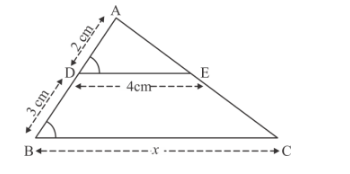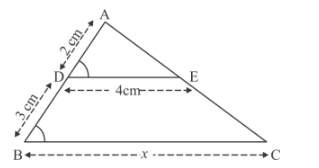# In Fig. 2, If DE || BC, then x equals`
Question:

In Fig. 2, If DE || BC, then x equals

(a) 6 cm

(b) 8 cm

(c) 10 cm

(d) 12.5 cmSolution:

In the given figure we have DE||BC. We have to find x.

In the following given figure DE||BC, so triangle ADE and triangle ABC are similar triangle.So we have the following relation

$\frac{B C}{D E}=\frac{A B}{A D}$

$\frac{x}{4}=\frac{3+2}{2}$

$\Rightarrow x=10$

Hence the correct option is